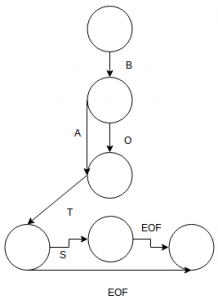51. Determine the longest string which is described by the given Directed Acyclic Word Graph.a. BATS b. BOATS c. BOT d. None of the mentioned

 52. Which of the following statements for a simple graph is correct? a. Every path is a trail b. Every trail is a path c. Every trail is a path as well as every path is a trail d. None of the mentioned
 Answer: (a).Every path is a trail

 53. What is the number of edges present in a complete graph having n vertices? a. (n*(n+1))/2 b. (n*(n-1))/2 c. n d. Information given is insufficient

 54. In a simple graph, the number of edges is equal to twice the sum of the degrees of the vertices. a. True b. False c. May be d. Can't say

 55. A connected planar graph having 6 vertices, 7 edges contains _____________ regions. a. 15 b. 3 c. 1 d. 11

 56. If a simple graph G, contains n vertices and m edges, the number of edges in the Graph G'(Complement of G) is ___________ a. (n*n-n-2*m)/2 b. (n*n+n+2*m)/2 c. (n*n-n-2*m)/2 d. (n*n-n+2*m)/2

 57. Which of the following properties does a simple graph not hold? a. Must be connected b. Must be unweighted c. Must have no loops or multiple edges d. All of the mentioned